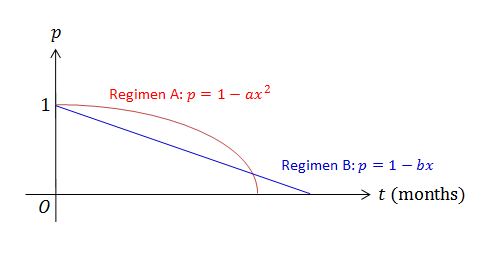Quantitative Finance

# Continuous Random Variables - Problem SolvingSuppose there are two new effective regimens (regimen $A$ and regimen $B$) that can be used for treating advanced pancreatic cancer. Dr. Johnson wants to decide which regimen to use for his patient. According to the national data of pancreatic cancer patients, the survival curves when using each regimen are as shown in the figure above. This data suggests that regimen $A$ results in a better survival rate. How much longer (in months) is the life expectancy when using regimen $A$ than that when administrating regimen $B$?

Details and Assumptions

• A survival curve plots the fraction of survived patients according to elapsed time.
• $a=\frac{1}{324}$ and $b=\frac{1}{23}.$
• The figure is not drawn to scale.

Suppose there is a bus stop where the bus stops every $7$ minutes. If Tom goes to that bus stop at an arbitrary time, what is the variance of the time Tom has to wait for the bus?

Let continuous random variables $X$ and $Y$ have the joint probability density function $f(x,y)=\frac{1}{9}xy$ for $0\leq x\leq2$ and $0\leq y\leq3.$ Find the conditional probability $P(X\leq1|Y\leq2).$

If the probability distributions of continuous random variables $X$ and $Y$ are defined in the domains $0 \le x \le 1$ and $0\le y\le1$ as follows: $f_X(x) = 6x^{5} , f_Y(y) = 10 y^{9},$ what is the covariance between $X$ and $Y ?$

Suppose that the life expectancy $X$ of a cat species has the probability density function $f(x)=kx(x-20)$ for $0\leq X\leq20.$ John the farmer decided to breed this species, and bought hundreds of these newborn cats. If John currently has $2000$ cats, all of which are $10$ years old, how many of these cats will die before becoming $14$ years old?

Note: Ignore reproduction of the cats.

×